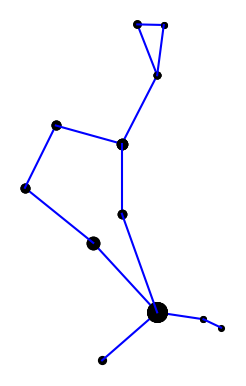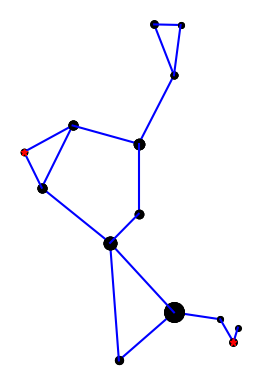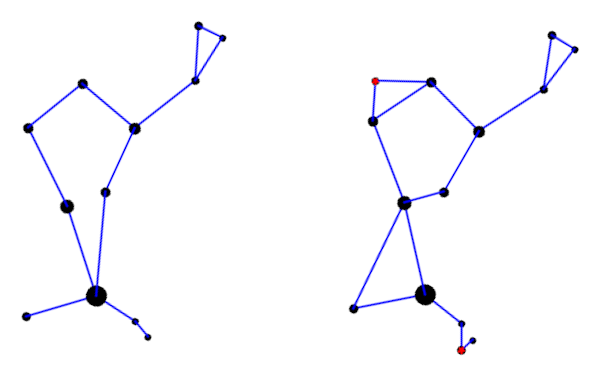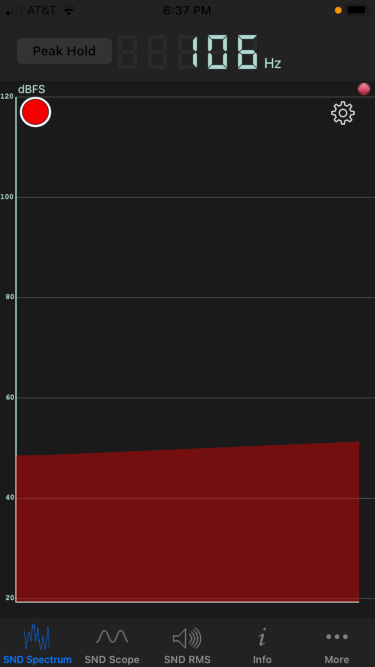# Connecting the dots differently

A couple weeks ago I wrote about how H. A. Rey introduced a new way of looking at the constellations, making them look more like their names. That post used Leo as an example. This post looks at Boötes (The Herdsman) .

Here is the constellation using the connections indicated in the IAU star chart.Here is the constellation using the connections drawn in Rey’s book .Rey’s version adds two stars, highlighted in red, but mostly connects the same stars in a different way. I suppose the herdsman is standing in the IAU version; it’s hard to tell. In Rey’s version, the huntsman is clearly seated and smoking a pipe. This is easier to see if we rotate the image a bit.Here’s a comparison of the two interpretations side-by-side.Here is the Python code that produced the two images. It’s a little cleaner than the code in the earlier post, and it draws larger dots to represent brighter stars.

import matplotlib.pyplot as plt

# data from https://en.wikipedia.org/wiki/List_of_stars_in_Bo%C3%B6tes

α = (14 + 16/60, 19 + 11/60, 0.0)
β = (15 +  2/60, 40 + 23/60, 3.5)
γ = (14 + 32/60, 38 + 18/60, 3.0)
δ = (15 + 16/60, 33 + 19/60, 3.5)
ε = (14 + 45/60, 27 +  4/60, 2.3)
ζ = (14 + 41/60, 13 + 44/60, 3.8)
η = (13 + 55/60, 18 + 24/60, 4.5)
θ = (14 + 25/60, 51 + 51/60, 4.0)
κ = (14 + 13/60, 51 + 47/60, 4.5)
λ = (14 + 16/60, 46 +  5/60, 4.2)
μ = (15 + 24/60, 37 + 23/60, 4.3)
υ = (13 + 49/60, 15 + 48/60, 4.0)
τ = (13 + 47/60, 17 + 27/60, 4.5)
ρ = (14 + 32/60, 30 + 22/60, 3.6)

k = -15 # reverse and scale horizontal axis

def plot_star(s, m):
plt.plot(k*s, s, m, markersize=14-2.2*s)

def join(s0, s1, m='ko'):
plot_star(s0, m)
plot_star(s1, m)

plt.plot([k*s0, k*s1], [s0, s1], 'b-')

def draw_iau():

join(α, η)
join(η, τ)
join(α, ζ)
join(α, ϵ)
join(ϵ, δ)
join(δ, β)
join(β, γ)
join(γ, λ)
join(λ, θ)
join(θ, κ)
join(κ, λ)
join(γ, ρ)
join(ρ, α)

def draw_rey():

join(α, η)
join(η, υ)
join(υ, τ)

join(α, ζ)
join(α, ϵ)
join(ζ, ϵ)

join(ϵ, δ)
join(δ, β)
join(δ, μ)
join(μ, β)
join(β, γ)
join(γ, λ)
join(λ, θ)
join(θ, κ)
join(κ, λ)
join(γ, ρ)
join(ρ, ϵ)

plot_star(μ, 'r*')
plot_star(υ, 'r*')
return

draw_iau()
plt.gca().set_aspect('equal')
plt.axis('off')
plt.savefig("bootes_iau.png")
plt.close()

draw_rey()
plt.gca().set_aspect('equal')
plt.axis('off')
plt.savefig("bootes_rey.png")
plt.close()


***

 The diaeresis over the second ‘o’ in Boötes means the two vowels are to be pronounced separately: bo-OH-tes. You may have seen the same pattern in Laocoön or oogenesis. The latter is written without a diaresis now, but I bet authors used to write it with a diaeresis on the second ‘o’.

 H. A. Rey. The Stars: A New Way to See Them, Second Edition.

# Plotting constellations

Suppose you wanted to write a program to plot constellations. This leads down some interesting rabbit trails.

When you look up data on stars in constellations you run into two meanings of constellation. For example, Leo is a region of the night sky containing an untold number of stars. It is also a pattern of nine particular stars connected by imaginary lines. It’s easier to find data on the former, say sorted by brightness.

Are the nine brightest stars in Leo-the-region the nine stars of Leo-the-stick-figure? Not exactly, but close.

Wikipedia has an article that list stars in each constellation region, and star charts that have constellations as stick figures. If the stars on the chart are labeled, you can cross reference them with Wikipedia.

On a particular star chart I have, the stars in Leo are labeled with their Bayer designation. Roughly speaking the Bayer designation labels the stars within a constellation with Greek letters in descending order of brightness, but there are inconsistencies. The nomenclature goes back to Johann Bayer (1572–1625) and has its flaws.

The stars in Leo, in line-drawing order, are

1. δ Leo
2. Denebola (β Leo)
3. θ Leo
4. Regulus (α Leo)
5. η Leo
6. γ Leo
7. ζ Leo
8. μ Leo
9. ε Leo

You can look up the coordinates of these stars here. Line-drawing order does not correspond to brightness order, so without a labeled star chart you’d have some research to do. My chart labels all the stars in Leo (the stick figure), but not, for example, in Virgo.

γ Leo is actually two stars, and Wikipedia ranks the brightness of the stars a little differently than Bayer did, which is understandable since brightness could not be objectively measured in his day. Wikipedia also inserts a few stars in between the stars listed above.

Here’s a plot of Leo using the data referenced above.# Helmholtz resonator revisited

We finished a bottle of wine this evening, and I blew across the top as I often do. (Don’t worry: I only do this at home. If we’re ever in a restaurant together, I won’t embarrass you by blowing across the neck of an empty bottle.)

The pitch sounded lower than I expected, so I revisited some calculations I did last year.

As I wrote about here, a wine bottle is approximately a Helmhotz resonator. The geometric approximation is not very good, but the pitch prediction usually is. An ideal Helmholtz resonator is a cylinder attached to a sphere, and a typical wine bottle is more like a cylinder attached to a larger cylinder. But the formula predicting pitch is robust to departures from ideal assumptions.

As noted before, the formula for the fundamental frequency of a Helmholtz resonator iswhere the variables are as follows:

• f, frequency in Hz
• v, velocity of sound
• A, area of the opening
• L, length of the neck
• V, volume

The opening diameter was 2 cm, the neck length 9 cm, and the volume 750 cm³. All these are typical. The predicted frequency is f = 118 Hz. The measured frequency was 106 Hz, measured by the Sonic Tools phone app.The actual frequency was about 10% lower than predicted. This is about a whole step lower in musical terms. I could certainly hear an interval that large if I heard the two pitches sequentially. But I don’t have perfect pitch, and so I’m skeptical whether I could actually notice a pitch difference of that size from memory.

I started a new Twitter account this morning: @ElementFact. I’m thinking the account will post things like scientific facts about each element but also some history around how the element was discovered and named and other lore associated with the element.We’ll see how this goes. I’ve started many Twitter accounts over the years. Some have taken off and some have not.

Six months ago I started @TensorFact as an experiment to see what would happen with a narrowly focused account. It did moderately well, but I ran out of ideas for content. This will be the last week for that account.

You can see a list of my current Twitter accounts here.

# Koide’s coincidence

The p-norm of a vector is defined to be the pth root of the sum of its pth powers.Such norms occur frequently in application . Yoshio Koide discovered in 1981 that if you take the masses of the electron, muon, and tau particles, the ratio of the 1 norm to the 1/2 norm is very nearly 2/3. Explicitly,to at least four decimal places. Since the ratio is tantalizingly close to 2/3, some believe there’s something profound going on here and the value is exactly 2/3, but others believe it’s just a coincidence.

The value of 2/3 is interesting for two reasons. Obviously it’s a small integer ratio. But it’s also exactly the midpoint between the smallest and largest possible value. More on that below.

Is the value 2/3 within the measure error of the constants? We’ll investigate that with a little Python code.

## Python code

The masses of particles are available in the physical_constants dictionary in scipy.constants. For each constant, the dictionary contains the best estimate of the value, the units, and the uncertainty (standard deviation) in the measurement .

    from scipy.constants import physical_constants as pc
from scipy.stats import norm

def pnorm(v, p):
return sum(x**p for x in v)**(1/p)

def f(v):
return pnorm(v, 1) / pnorm(v, 0.5)

m_e = pc["electron mass"]
m_m = pc["muon mass"]
m_t = pc["tau mass"]

v0 = [m_e, m_m, m_t]

print(f(v0))


This says that the ratio of the 1 norm and 1/2 norm is 0.666658, slightly less than 2/3. Could the value be exactly 2/3 within the resolution of the measurements? How could we find out?

## Measurement uncertainty

The function f above is minimized when its arguments are all equal, and maximized when its arguments are maximally unequal. To see this, note that f(1, 1, 1) = 1/3 and f(1, 0, 0) = 1. You can prove that those are indeed the minimum and maximum values. To see if we can make f larger, we want to increase the largest value, the mass of tau, and decrease the others. If we move each value one standard deviation in the desired direction, we get

    v1 = [m_e - m_e,
m_m - m_m,
m_t + m_t]
print(f(v1))


which returns 0.6666674, just slightly bigger than 2/3. Since the value can be bigger than 2/3, and less than 2/3, the intermediate value theorem says there are values of the constants within one standard deviation of their mean for which we get exactly 2/3.

Now that we’ve shown that it’s possible to get a value above 2/3, how likely is it? We can do a simulation, assuming each measurement is normally distributed.

    N = 1000
above = 0
for _ in range(N):
r_e = norm(m_e, m_e).rvs()
r_m = norm(m_m, m_m).rvs()
r_t = norm(m_t, m_t).rvs()
t = f([r_e, r_m, r_t])
if t > 2/3:
above += 1
print(above)


When we I ran this, I got 168 values above 2/3 and the rest below. So based solely on our calculations here, not taking into account any other information that may be important, it’s plausible that Koide’s ratio is exactly 2/3.

***

 Strictly speaking, we should take the absolute values of the vector components. Since we’re talking about masses here, I simplified slightly by assuming the components are non-negative.

Also, what I’m calling the p “norm” is only a norm if p is at least 1. Values of p less than 1 do occur in application, even though the functions they define are not norms. I’m pretty sure I’ve blogged about such an application, but I haven’t found the post.

 The SciPy library obtained its values for the constants and their uncertainties from the CODATA recommended values, published in 2014.

# Linear regression and planet spacing

A while back I wrote about how planets are evenly spaced on a log scale. I made a bunch of plots, based on our solar system and the extrasolar systems with the most planets, and said noted that they’re all roughly straight lines. Here’s the plot for our solar system, including dwarf planets, with distance on a logarithmic scale.This post is a quick follow up to that one. You can quantify how straight the lines are by using linear regression and comparing the actual spacing with the spacing given by the best straight line. Here I’m regressing the log of the distance of each planet from its star on the planet’s ordinal position.

NB: I am only using regression output as a measure of goodness of fit. I am not interpreting anything as a probability.

|-----------+-----------+----------+-----------|
| System    | Adjusted  | Slope    | Intercept |
|           | R-squared | p-value  | p-value   |
|-----------+-----------+----------+-----------|
| home      |    0.9943 | 1.29e-11 |  2.84e-08 |
| kepler90  |    0.9571 | 1.58e-05 |  1.29e-06 |
| hd10180   |    0.9655 | 1.41e-06 |  2.03e-07 |
| hr8832    |    0.9444 | 1.60e-04 |  5.57e-05 |
| trappist1 |    0.9932 | 8.30e-07 |  1.09e-09 |
| kepler11  |    0.9530 | 5.38e-04 |  2.00e-05 |
| hd40307   |    0.9691 | 2.30e-04 |  1.77e-05 |
| kepler20  |    0.9939 | 8.83e-06 |  3.36e-07 |
| hd34445   |    0.9679 | 2.50e-04 |  4.64e-04 |
|-----------+-----------+----------+-----------|


R² is typically interpreted as how much of the variation in the data is explained by the model. In the table above, the smallest value of R² is 94%.

p-values are commonly, and wrongly, understood to be the probability of a model assumption being incorrect. As I said above, I’m completely avoiding any interpretation of p-values as the probability of anything, only noting that small values are consistent with a good fit.

Journals commonly, and wrongly, are willing to assume that anything with a p-value less than 0.05 is probably true. Some are saying the cutoff should be 0.005. There are problems with using any p-value cutoff, but I don’t want to get into here. I’m only saying that small p-values are typically seen as evidence that a model fits, and the values above are orders of magnitude smaller than what journals consider acceptable evidence.

When I posted my article about planet spacing I got some heated feedback saying that this isn’t exact, that it’s unscientific, etc. I thought that was strange. I never said it was exact, only that it was a rough pattern. And although it’s not exact, it would be hard to find empirical studies of anything with such a good fit. If you held economics or psychology, for example, to the same standards of evidence, there wouldn’t be much left.

This pattern is known as the Titius-Bode law. I stumbled on it by making some plots. I assumed from the beginning that someone else must have done the same exercise and that the pattern had a name, but I didn’t know that name until later.

Someone sent me a paper that analyzes the data on extrasolar planets and Bode’s law, something much more sophisticated than the crude sketch above, but unfortunately I can’t find it this morning. I don’t recall what they did. Maybe they fit a hierarchical model where each system has its own slope and intercept.

One criticism has been that by regressing against planet order, you automatically get a monotone function. That’s true, but you do get a much better fit on a log scale than on a linear scale in any case. You might look at just the relative planet spacings without reference to order.

# Proving life exists on Earth

NASA’s Galileo mission was primarily designed to explore Jupiter and its moons. In 1989, the Galileo probe started out traveling away from Jupiter in order to do a gravity assist swing around Venus. About a year later it also did a gravity assist maneuver around Earth. Carl Sagan suggested that when passing Earth, the Galileo probe should turn its sensors on Earth to look for signs of life.Now obviously we know there’s life on Earth. But if we’re going look for life on other planets, it’s reasonable to ask that our methods return positive results when examining the one planet we know for sure does host life. So scientists looked at the data from Galileo as if it were coming from another planet to see what patterns in the data might indicate life.

I’ve started using looking for life on Earth as a metaphor. I’m working on a project right now where I’m looking for a needle in a haystack, or rather another needle in a haystack: I knew that one needle existed before I got started. So I want to make sure that my search procedure at least finds the one positive result I already know exists. I explained to a colleague that we need to make sure we can at least find life on Earth.

This reminds me of simulation work. You make up a scenario and treat it as the state of nature. Then pretend you don’t know that state, and see how successful your method is at discovering that state. It’s sort of a schizophrenic way of thinking, pretending that half of your brain doesn’t know what the other half is doing.

It also reminds me of software testing. The most trivial tests can be surprisingly effective at finding bugs. So you write a few tests to confirm that there’s life on Earth.

### Related posts

 I found out about Galileo’s Earth reconnaissance listening to the latest episode of the .NET Rocks! podcast.

# Planets evenly spaced on log scale

The previous post was about Kepler’s observation that the planets were spaced out around the sun the same way that nested regular solids would be. Kepler only knew of six planets, which was very convenient because there are only five regular solids. In fact, Kepler thought there could only be six planets because there are only five regular solids.

The distances to each of the planets is roughly a geometric series. Ratios of consecutive distances are between 1.3 and 3.4. That means the distances should be fairly evenly spaced on a logarithmic scale. The plot below shows that this is the case.The plot goes beyond the six planets known in Kepler’s day and adds four more: Uranus, Neptune, Pluto, and Eris. Here I’m counting the two largest Kuiper belt objects as planets. Distances are measured in astronomical units, i.e. Earth = 1.

Update: The fit is even better if we include Ceres, the largest object in the asteroid belt. It’s now called a dwarf planet, but it was considered a planet for the first fifty years after it was discovered.## Extrasolar planets

Is this just true of our solar system, or is it true of other planetary systems as well? At the time of writing, we know of one planetary system outside our own that has 8 planets. Three other systems have around 7 planets; I say “around” because it depends on whether you include unconfirmed planets. The spacing between planets in these other systems is also fairly even on a logarithmic scale. Data on planet distances is taken from each system’s Wikipedia page. Distances are semimajor axes, not average distances.

This post just shows plots. See this follow up post for regression results.

### Kepler-90

Kepler-90 is the only planetary system outside our own with eight confirmed planets that we know of.### HD 10180

HD 10180 has seven confirmed planets and two unconfirmed planets. The unconfirmed planets are included below, the 3rd and 6th objects.### HR 8832

The next largest system is HR 8832 with five confirmed planets and two unconfirmed, numbers 5 and 6 below. It would work out well for this post if the 6th object were found to be a little closer to its star.### TRAPPIST-1

TRAPPIST-1 is interesting because the planets are very close to their star, ranging from 0.01 to 0.06 AU. Also, this system follows an even logarithmic spacing more than the others.#### Systems with six planets

There are currently four known systems with four planets: Kepler-11, Kepler-20, HD 40307, and HD 34445. They also appear to have planets evenly spaced on a log scale.Like TRAPPIST-1, Kepler-20 has planets closer in and more evenly spaced (on a log scale).

# Mercury and the bandwagon effectThe study of the planet Mercury provides two examples of the bandwagon effect. In her new book Worlds Fantastic, Worlds Familiar, planetary astronomer Bonnie Buratti writes

The study of Mercury … illustrates one of the most confounding bugaboos of the scientific method: the bandwagon effect. Scientists are only human, and they impose their own prejudices and foregone conclusions on their experiments.

Around 1800, Johann Schroeter determined that Mercury had a rotational period of 24 hours. This view held for eight decades.

In the 1880’s, Giovanni Schiaparelli determined that Mercury was tidally locked, making one rotation on its axis for every orbits around the sun. This view also held for eight decades.

In 1965, radar measurements of Mercury showed that Mercury completes 3 rotations in every 2 orbits around the sun.

Studying Mercury is difficult since it is only visible near the horizon and around sunrise and sunset, i.e. when the sun’s light interferes. And it is understandable that someone would confuse a 3:2 resonance with tidal locking. Still, for two periods of eight decades each, astronomers looked at Mercury and concluded what they expected.

The difficulty of seeing Mercury objectively was compounded by two incorrect but satisfying metaphors. First that Mercury was like Earth, rotating every 24 hours, then that Mercury was like the moon, orbiting the sun the same way the moon orbits Earth.

Buratti mentions the famous Millikan oil drop experiment as another example of the bandwagon effect.

… Millikan’s value for the electron’s charge was slightly in error—he had used a wrong value for the viscosity of air. But future experimenters all seemed to get Millikan’s number. Having done the experiment myself I can see that they just picked those values that agreed with previous results.

Buratti explains that Millikan’s experiment is hard to do and “it is impossible to successfully do it without abandoning most data.” This is what I like to call acceptance-rejection modeling.

The name comes from the acceptance-rejection method of random number generation. For example, the obvious way to generate truncated normal random values is to generate (unrestricted) normal random values and simply throw out the ones that lie outside the interval we’d like to truncate to. This is inefficient if we’re truncating to a small interval, but it always works. We’re conforming our samples to a pre-determined distribution, which is OK when we do it intentionally. The problem comes when we do it unintentionally.Photo of Mercury above via NASA

# Freudian hypothesis testingIn his paper Mindless statistics, Gerd Gigerenzer uses a Freudian analogy to describe the mental conflict researchers experience over statistical hypothesis testing. He says that the “statistical ritual” of NHST (null hypothesis significance testing) “is a form of conflict resolution, like compulsive hand washing.”

In Gigerenzer’s analogy, the id represents Bayesian analysis. Deep down, a researcher wants to know the probabilities of hypotheses being true. This is something that Bayesian statistics makes possible, but more conventional frequentist statistics does not.

The ego represents R. A. Fisher’s significance testing: specify a null hypothesis only, not an alternative, and report a p-value. Significance is calculated after collecting the data. This makes it easy to publish papers. The researcher never clearly states his hypothesis, and yet takes credit for having established it after rejecting the null. This leads to feelings of guilt and shame.

The superego represents the Neyman-Pearson version of hypothesis testing: pre-specified alternative hypotheses, power and sample size calculations, etc. Neyman and Pearson insist that hypothesis testing is about what to do, not what to believe. 

I assume Gigerenzer doesn’t take this analogy too seriously. In context, it’s a humorous interlude in his polemic against rote statistical ritual.

But there really is a conflict in hypothesis testing. Researchers naturally think in Bayesian terms, and interpret frequentist results as if they were Bayesian. They really do want probabilities associated with hypotheses, and will imagine they have them even though frequentist theory explicitly forbids this. The rest of the analogy, comparing the ego and superego to Fisher and Neyman-Pearson respectively, seems weaker to me. But I suppose you could imagine Neyman and Pearson playing the role of your conscience, making you feel guilty about the pragmatic but unprincipled use of p-values.

* * *

 “No test based upon a theory of probability can by itself provide any valuable evidence of the truth or falsehood of a hypothesis. But we may look at the purpose of tests from another viewpoint. Without hoping to know whether each separate hypothesis is true or false, we may search for rules to govern behaviour in regard to them, in following which we insure that, in the long run of experience, we shall not often be wrong.”

Neyman J, Pearson E. On the problem of the most efficient tests of statistical hypotheses. Philos Trans Roy Soc A, 1933;231:289, 337.